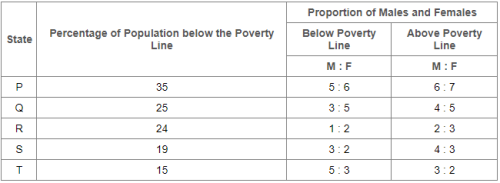Courses

# Can you explain the answer of this question below:The following table gives the percentage distribution of population of five states, P, Q, R, S and T on the basis of poverty line and also on the basis of sex.If the population of males below poverty line for State Q is 2.4 million and that for State T is 6 million, then the total populations of States Q and T are in the ratio?A:2:5B:1:3C:3:7D:4:9The answer is a. Related Test: Practice Test: Table Charts - 1

## LR QuestionManoj Yadav May 22, 2018
For State Q:Male population below poverty line = 2.4 million.Let the female population below poverty line be x million.Then, 3 : 5 = 2.4 : x => x = 5 x 2.4/3 = 4.Therefore Total population below poverty line = (2.4 + 4) = 6.4 million.If Nq be the total population of State Q, then,25% of Nq = 6.4 million => Nq = (6.4 x 100)/25 million = 25.6 million.For State T:Male population below poverty line = 6 million.Let the female population below poverty line be y million.Then, 5 : 3 = 6 : y => y = 3 x 6/5 = 3.6.Therefore Total population below poverty line = (6 + 3.6) = 9.6 million.If Nt be the total population of State T, then,15% of Nt = 9.6 million => Nt = (9.6 x 100)/15 million = 64 million.Thus, Required ratio = Nq/Nt = 25.6/64 = 0.4 = 2/5.

This discussion on Can you explain the answer of this question below:The following table gives the percentage distribution of population of five states, P, Q, R, S and T on the basis of poverty line and also on the basis of sex.If the population of males below poverty line for State Q is 2.4 million and that for State T is 6 million, then the total populations of States Q and T are in the ratio?A:2:5B:1:3C:3:7D:4:9The answer is a. is done on EduRev Study Group by LR Students. The Questions and Answers of Can you explain the answer of this question below:The following table gives the percentage distribution of population of five states, P, Q, R, S and T on the basis of poverty line and also on the basis of sex.If the population of males below poverty line for State Q is 2.4 million and that for State T is 6 million, then the total populations of States Q and T are in the ratio?A:2:5B:1:3C:3:7D:4:9The answer is a. are solved by group of students and teacher of LR, which is also the largest student community of LR. If the answer is not available please wait for a while and a community member will probably answer this soon. You can study other questions, MCQs, videos and tests for LR on EduRev and even discuss your questions like Can you explain the answer of this question below:The following table gives the percentage distribution of population of five states, P, Q, R, S and T on the basis of poverty line and also on the basis of sex.If the population of males below poverty line for State Q is 2.4 million and that for State T is 6 million, then the total populations of States Q and T are in the ratio?A:2:5B:1:3C:3:7D:4:9The answer is a. over here on EduRev! Apart from being the largest LR community, EduRev has the largest solved Question bank for LR.